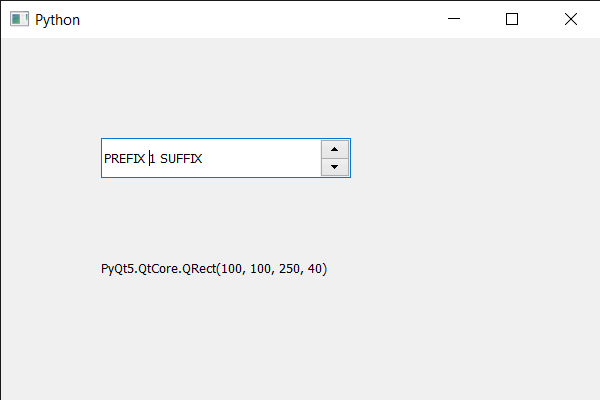PyQt5 QSpinBox – Getting current geometry

• Last Updated : 22 Jun, 2021

In this article we will see how we can get the current geometry of the spin box. Geometry is basically the position as well as the size of the spin box. Setting geometry to the spin box can change its size as well as its position. We use setGeometry method to set or change the geometry of the spin box.
In order to do this we use geometry method with the spin box object.

Syntax : font_metrics.geometry()
Argument : It takes no argument
Return : It returns QRect object

Below is the implementation

Python3

 # importing librariesfrom PyQt5.QtWidgets import *from PyQt5 import QtCore, QtGuifrom PyQt5.QtGui import *from PyQt5.QtCore import *import sys  class Window(QMainWindow):     def __init__(self):        super().__init__()         # setting title        self.setWindowTitle("Python ")         # setting geometry        self.setGeometry(100, 100, 600, 400)         # calling method        self.UiComponents()         # showing all the widgets        self.show()         # method for widgets    def UiComponents(self):        # creating spin box        self.spin = QSpinBox(self)         # setting geometry to spin box        self.spin.setGeometry(100, 100, 250, 40)         # setting range to the spin box        self.spin.setRange(1, 999999)         # setting prefix to spin        self.spin.setPrefix("PREFIX ")         # setting suffix to spin        self.spin.setSuffix(" SUFFIX")          # creating a label        label = QLabel(self)         # making label multi line        label.setWordWrap(True)         # setting geometry to the label        label.setGeometry(100, 200, 300, 60)         # getting the current geometry        current_geo = self.spin.geometry()         # setting text to the label        label.setText(str(current_geo))  # create pyqt5 appApp = QApplication(sys.argv) # create the instance of our Windowwindow = Window() # start the appsys.exit(App.exec())

Output :My Personal Notes arrow_drop_up